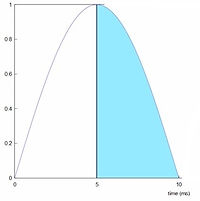## Pulse windows

Square gated sine wave carrier frequency? Sounds complicated right?PEMF pulses are applied in pulses per second. At the right is an example of 3 pulses per second = 3 Hz.

Some devices do just that: giving 3 pulses, which can be simple square waves (as in the picture) or triangle waves or sinus waves. On page 'Pulse forms' it was already elaborated on pulse forms and concluded that sinus waves are preferable but how can we obtain a fast 'Speed of induction' (= slew rate) if we use a sine wave? Inherent to the form of a sine wave it can never match the square wave, right?

And here comes the square gated pulse window: Open a square wave 'time window' duringwhich sine waves will pass. Now look at the same picture on the right and imagine that the orange area's are actually filled with sine waves! The time window simply opens a 'gate' through which these sine waves pass while the 'gate' is open and the gate is only open during the orange time! The sine waves are the actual energy carrier waves because they contain the pulsed electromagnetic energy.

BTW the above square time window is controlled by a very fast electronic switch.

OK but now the next question is: "But the sine waves inside these orange area's are still sine waves with slow rise times so where is the fast 'speed of induction'?"

In the left picture you see the Curatron half PEMF sine wave.

Notice that the actual sine wave starts at the left bottom corner but only when the maximum is reached the energy is allowed to pass though the gate towards the coils in the applicator, which is the blue area.

It is not difficult to see now that the speed of induction (= slew rate) is superb because this is where the blue area starts!So what happens now if the PEMF does not want to utilize the maximum intensity the Curatron unit is able to generate but we still want the fast speed of induction, right?

If you look at the picture on the right you see how the Curatron does this: it 'simply' lets the energy pass at later stage during the sine wave and we still have the superb PEMF slew rate where the blue area starts.